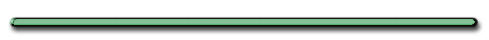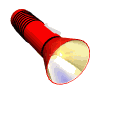HomeAccording to Einstein:

 Emax = hf - Wo eV or J eV or J eV or J

wo = work function - work to pull away electron

(different for each metal)

hf = Energy of light used

Emax = Energy of photoelectron (Max KE given to electron)

Electronvolt - very small energy unit:
1 eV = 1.6 X 10-19 J

Einstein uses

y = mx + b

to get his equation!Emax = hf - Wo eV or J eV or J eV or J

2. Threshold frequency - minimum frequency needed to expel electrons

Explained by Einstein

 Light Above Threshold Frequency Light Below Threshold  Frequency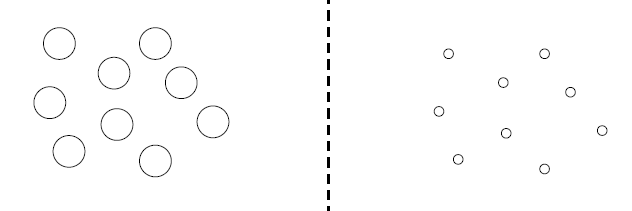(Strong enough to emit electrons) (Too weak to emit electrons)

Typical plot of Frequency vs. Emax (Maximum KE of photoelectron)Frequency

Threshold frequency of:

Metal A?

fo = 1.0 X 1014 Hz

Metal B?Frequency

fo = 2.5 X 1014 Hz

Metal C?Frequency

fo = 3.75 x 1014 Hz

Which metal has the
greatest work function?Frequency

Work Function - energy needed to pull away electron from atom.

 *  Wo = hfo  *

h = Planck's Constant

p.1 Reference

Ex 1) If a metal would only emit electrons when exposed to high frequency light what does that tell you about its work function?

Work function must be high

Ex) If the work function (Wo) of particular metal = 5.0 x 10-19 J Find the threshold frequency (fo)

( h = 6.63 x 10-34 Js )

Wo = hfo

fo = w/h

= (5 x 10-19 J/(6.63 x 10-34 Js)

fo = 7.5 x 1014 Hz

Since KEmax= hf - wo

Then …

 KE max= hf - hfo

(Use equation if given only frequencies)

PHOTON BY PHOTON DEVELOPMENT
OF A PHOTOGRAPH -Ref: P.G. Hewitt, Conceptual Physics, 9th ed.©2002 Pearson Education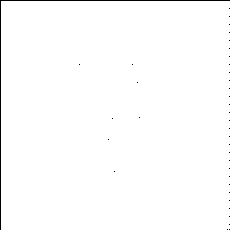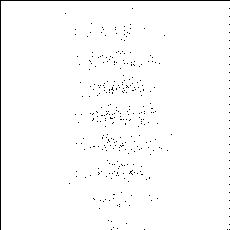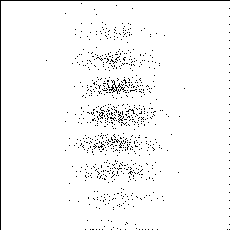Optics Research Group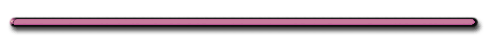Photon - Particle Collision